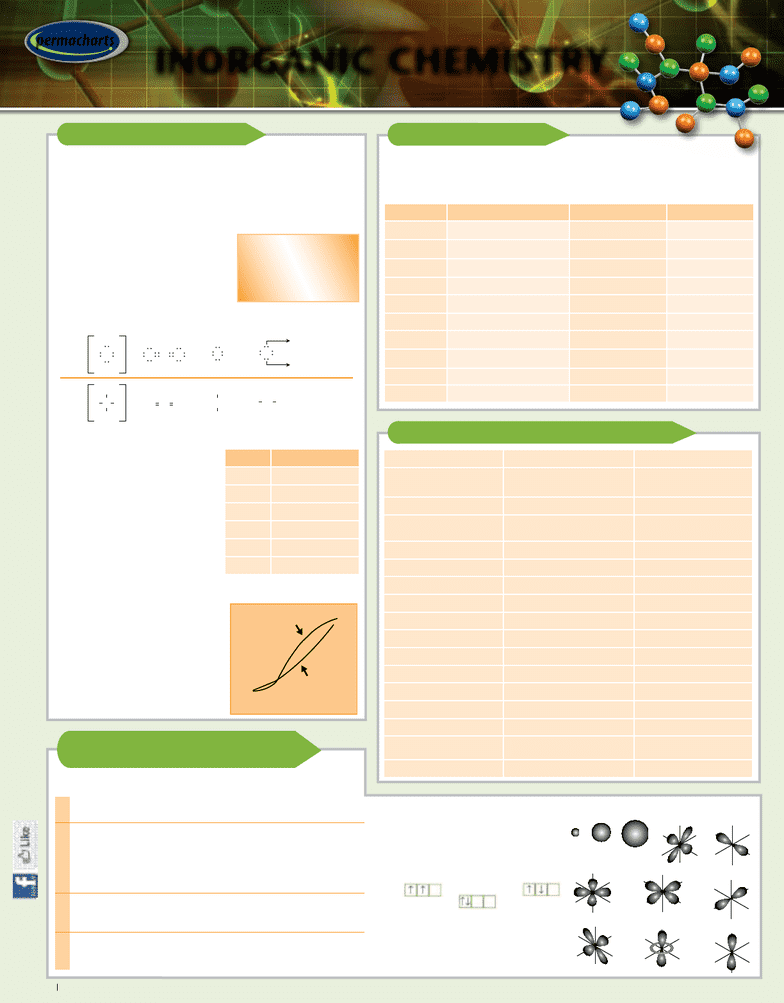Class Notes (1,100,000)
US (490,000)
Chicago (900)
CHEM (80)
All (2)
Reference Guide

# Inorganic Chemistry - Reference Guides

This preview shows page 1. to view the full 4 pages of the document.1INORGANIC CHEMISTRY 1-55080-833-8
IONIC BONDS
Atoms with low ionization energy form cations (+), and atoms
with high electron affinity form anions (–); attraction between
opposite charges on cations and ions creates an ionic bond
THE LEWIS OCTET RULE: COVALENT BONDS
Atoms other than hydrogen
(stable with 2 valence electrons)
will form bonds until they are
surrounded by 8 valence electrons
and have attained stable noble gas
configuration
In a single covalent bond, a pair of electrons is shared between
2 atoms; in multiple covalent bonds, 2 or 3 pairs are shared
BOND DISSOCIATION ENERGIES: CALCULATING ΔHr
ΔHr = Bond energies of reactants
Bond energies of products
Given H2 + Cl2 g2HCl, find the
enthalpy of reaction, rHr:
rHr = 436.4 + 242.7 – 2 x 431.9 =
184.7 kj/mol (exothermic reaction)
Notes: Exothermic = energy
(heat) flows out of system
• Endothermic = energy (heat)
flows into system
PERCENT IONIC CHARACTER OF A CHEMICAL BOND
Bonds with 50% or greater
ionic character are ionic;
bonds with less than 50%
ionic character are covalent
Transition from ionic to
covalent binding results in a
reduction in electrical
conductance, melting point,
and boiling point
CHEMICAL BONDING
TM
permacharts
INORGANIC CHEMISTRY
INTRODUCTION
The study of compounds devoid of carbon, except for CO, CO2, CS2, and
compounds containing CN, CO3
2–, and HCO3
INORGANIC COMPOUNDS
COMMON INORGANIC IONS
ELECTRONIC CONFIGURATION
OF THE ELEMENTS
Name Formula Name Formula
Alumina Al2O3Glauber’s Salt Na2SO4
Ammonia NH3Gypsum CaSO4•2H2O
Anatase TiO2Heavy Water D2O
Baking Soda NaHCO3Litharge PbO
Borax Na2B4O7(OH)4•8H2OMilk of Magnesia Mg(OH)2
Calcite CaCO3Quicklime CaO
Calomel Hg2Cl2Silica (SiO2)n
Cryolite Na3AlF6Slaked Lime Ca(OH)2
Dry Ice CO2 (solid) Table Salt NaCl
Galena PbS Washing Soda Na2CO3•10H2O
Aluminum (Al3+)Mercury (I), Mercurous (Hg2
2+)Hydride (H)
Ammonium (NH4
+)Mercury (II), Mercuric (Hg2+)Hydrogen Carbonate or
Bicarbonate (HCO3
)
Barium (Ba2+)Potassium (K+)Hydrogen Phosphate (HPO4
2)
(HSO4
)
Calcium (Ca2+)Sodium (Na+)Hydroxide (OH)
Cesium (Cs+)Strontium (Sr2+)Iodide (I)
Chromium (III) (Cr3+)Tin (II), Stannous (Sn2+)Nitrate (NO3
)
Cobalt (II), Cobaltous (Co2+)Zinc (Zn2+)Nitride (N3–)
Copper (I), Cuprous (Cu+)Bromide (Br)Nitrite (NO2
)
Copper (II), Cupric (Cu2+)Carbonate (CO3
2–)Oxide (O2–)
Hydrogen (H+)Chlorate (CIO3
)Permanganate (MnO4
)
Iron (II), Ferrous (Fe2+)Chloride (Cl)Peroxide (O2
2–)
Iron (III), Ferric (Fe3+)Chromate (CrO4
2–)Phosphate (PO4
3–)
Lead (II), Plumbous (Pb2+)Cyanide (CN)Sulfate (SO4
2–)
Lithium (Li+)Dichromate (Cr2O7
2–)Sulfide (S2–)
Manganese (II) (Mn2+)Dihydrogen Phosphate (H2PO4
)Sulfite (SO3
2–)
Magnesium (Mg2+)Flouride (F)Thiocyanate (SCN)
Bond Energy (kj/mol)
H – H 436.4
H – O 460
H – Cl 431.9
Cl – Cl 242.7
C – H 414
C – C 347
The Lewis Dot Diagram:
Consists of one dot for
each valence electron
surrounding the symbol
of the element
CO
O
H
H
H
N
H
H
O
CO
O
H
H
H
N
H
H
O
or
Lone or
non-bonding
electron pairs
3s
2s
1s
3dxy
z
yx
3d
z
yx
x
2
-y
2
z
yx
3dxz 2pz
z
yx
z
yx
3dyz
2p
x
z
yx
2py
z
yx
z
yx
3d
z
2
ORBITAL TYPES & SHAPES
100
80 -
60 -
40 -
20 -
0
% Ionic Character (IC x 100)
L. Pauling
IC=1–exp (–1
/
4
E2)
Hannay & Smyth
IC=0.16E+0.035E2
Difference in Electronegativity (E)
1
-
2
-
3
-
QUANTUM NUMBER & PROPERTIES
n Principal Quantum Number (n = 1, 2, 3, 4,…)
Determines size of electron orbital (roughly proportional to n2)
I Angular Momentum Quantum Number (I = 0, 1, 2,…, (n–1)
Determines shape of electron orbital
I = 0 Shape = spherical Designation = s (total 2e)
I = 1 Shape = lobes Designation = p (total 6e)
I = 2 Shape = lobes & donuts Designation = d (total 10e)
m Magnetic Quantum Number (m = 0 for I = 0, m = –1, 0, 1
for I = 1, m = –2, –1, 0, 1, 2 for I = 2) • Determines
orientation of electron orbital
ms Spin Quantum Number (ms = + ½ or – ½ for each n, l, m)
• Determines spin of electron • Unpaired (parallel) spin
produces paramagnetism • Parallel spin produces diamagnetism
HUND’S RULE
When more than one orbital
has the same energy, electrons
occupy separate orbitals, and do
so with parallel spins
U s i n g 2 p o r b i t a l s t o d e m o n s t r a t e :
is preferred over
or
PAULI’S EXCLUSION PRINCIPLE
No 2 electrons in an atom can have the
same 4 quantum numbers; no more
than 2 electrons can occupy any 1 orbital
H
H
H H
B
H
H
H H
B